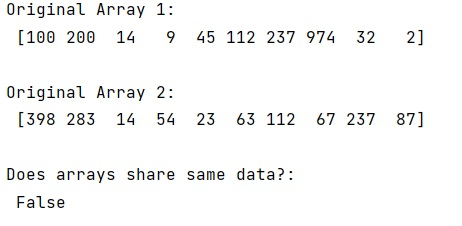# Is there a way to check if NumPy arrays share the same data?

Learn, how to check if NumPy arrays share the same data in Python?
Submitted by Pranit Sharma, on February 20, 2023

NumPy is an abbreviated form of Numerical Python. It is used for different types of scientific operations in python. Numpy is a vast library in python which is used for almost every kind of scientific or mathematical operation. It is itself an array which is a collection of various methods and functions for processing the arrays.

## Checking if NumPy arrays share the same data

Suppose that we are given two numpy arrays a and b and we need to check whether the two arrays share some data or not. Suppose that b is not a copy of a and there is just created some new meta-data attached to the same memory buffer that a is using, we need to find a way to check if two arrays reference the same memory buffer.

For this purpose, we can use the base attribute to check if an array shares the memory with another array. It is an attribute of numpy ndarray and its base object if memory is from some other object.

Let us understand with the help of an example,

## Python code to check if NumPy arrays share the same data

```# Import numpy
import numpy as np

# Creating two numpy arrays
arr1 = np.array([100, 200, 14, 9, 45, 112, 237, 974, 32, 2])
arr2 = np.array([398, 283, 14, 54, 23, 63,112 , 67, 237 , 87])

# Display original arrays
print("Original Array 1:\n",arr1,"\n")
print("Original Array 2:\n",arr2,"\n")

# Checking if arr1 is base of arr2
res = arr2.base is arr1

# Display Result
print("Does arrays share same data?:\n",res)
```

Output: